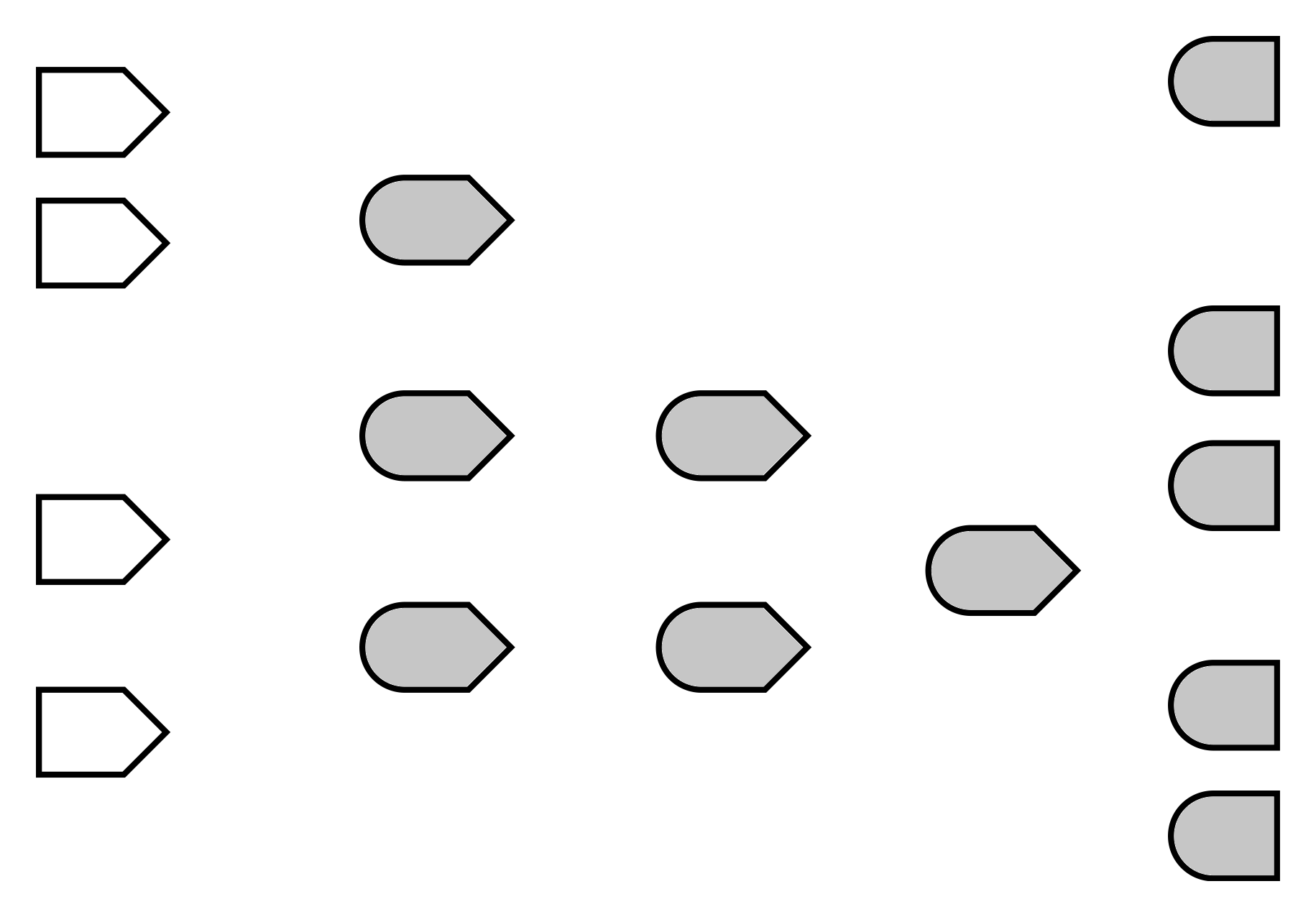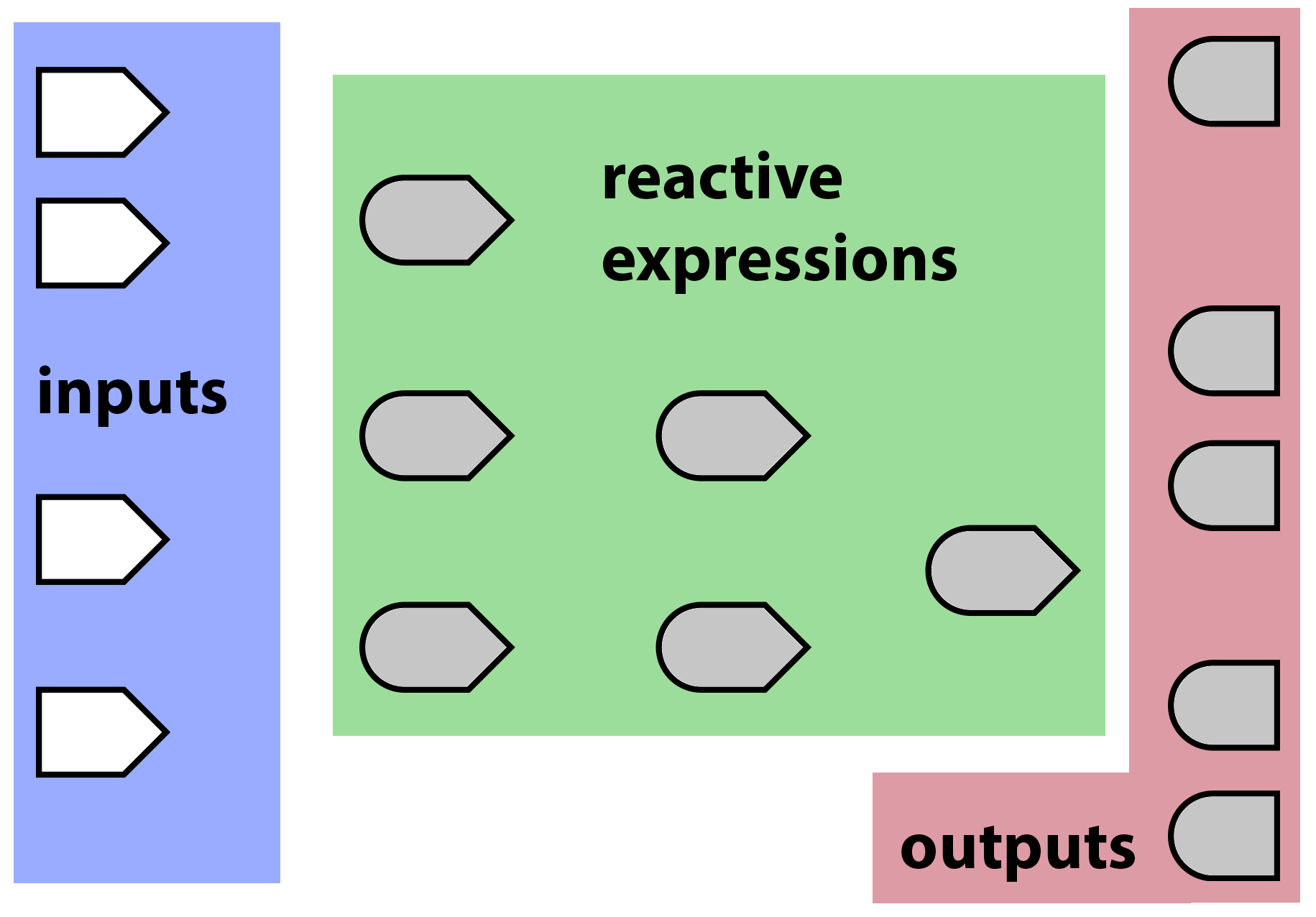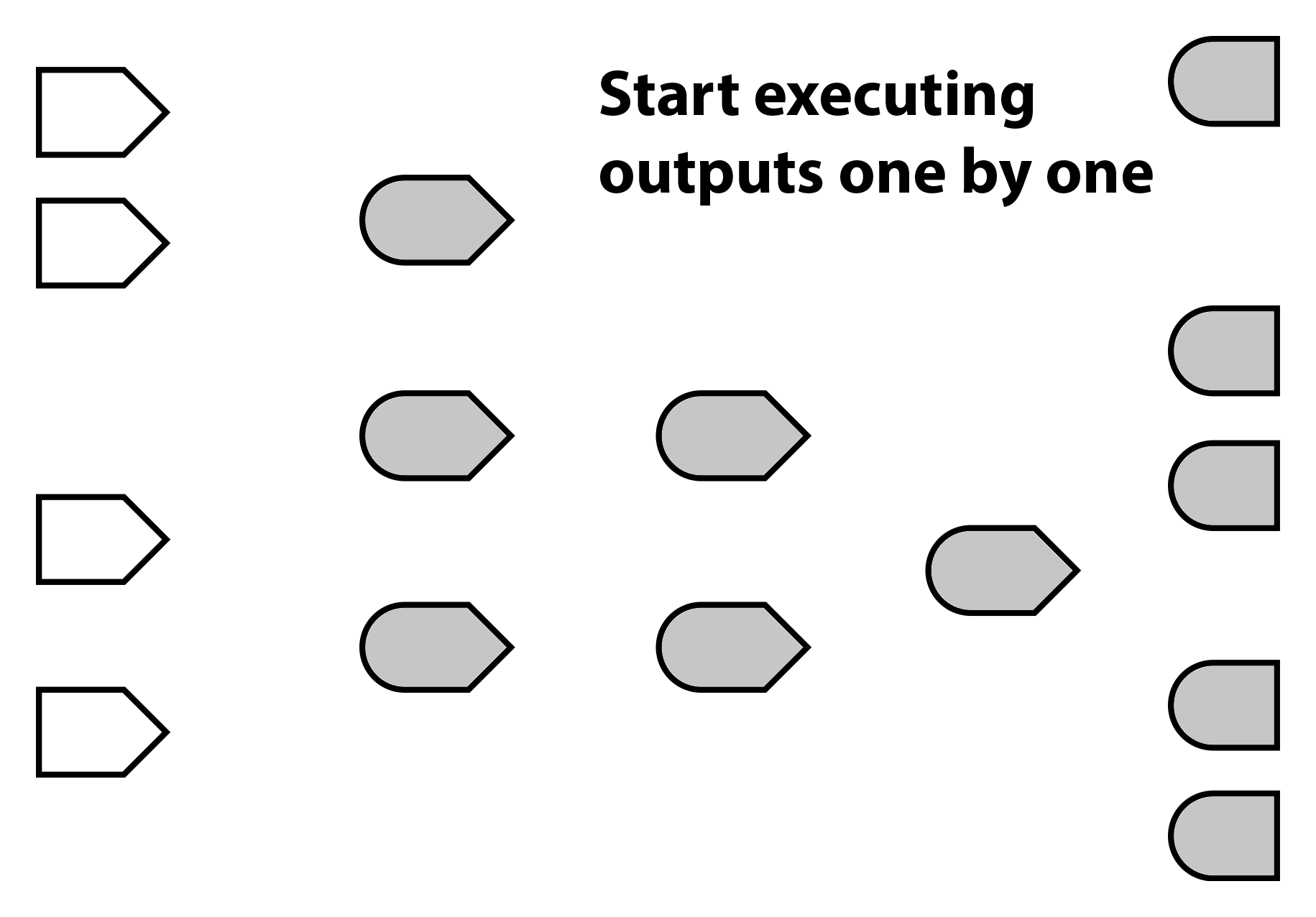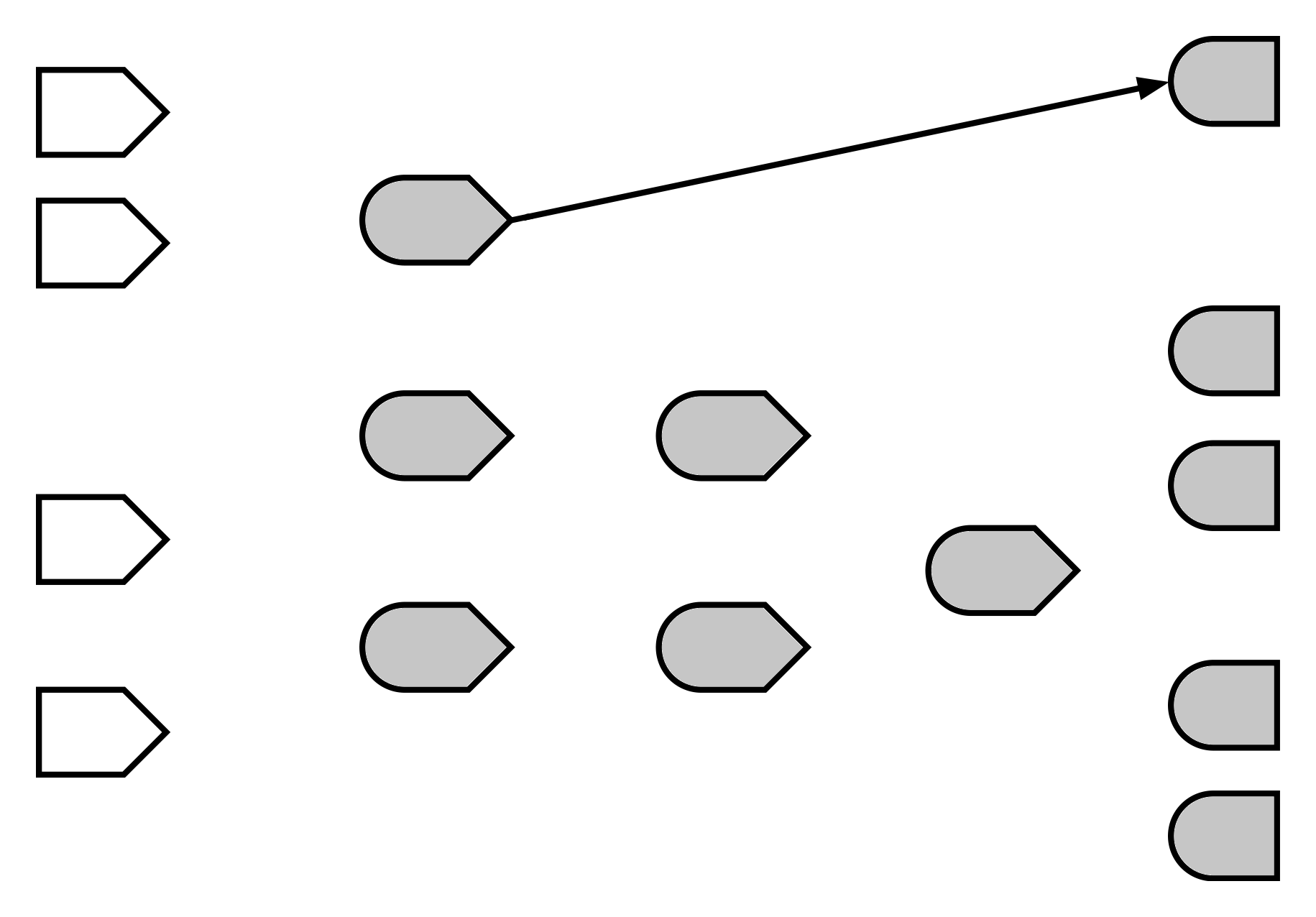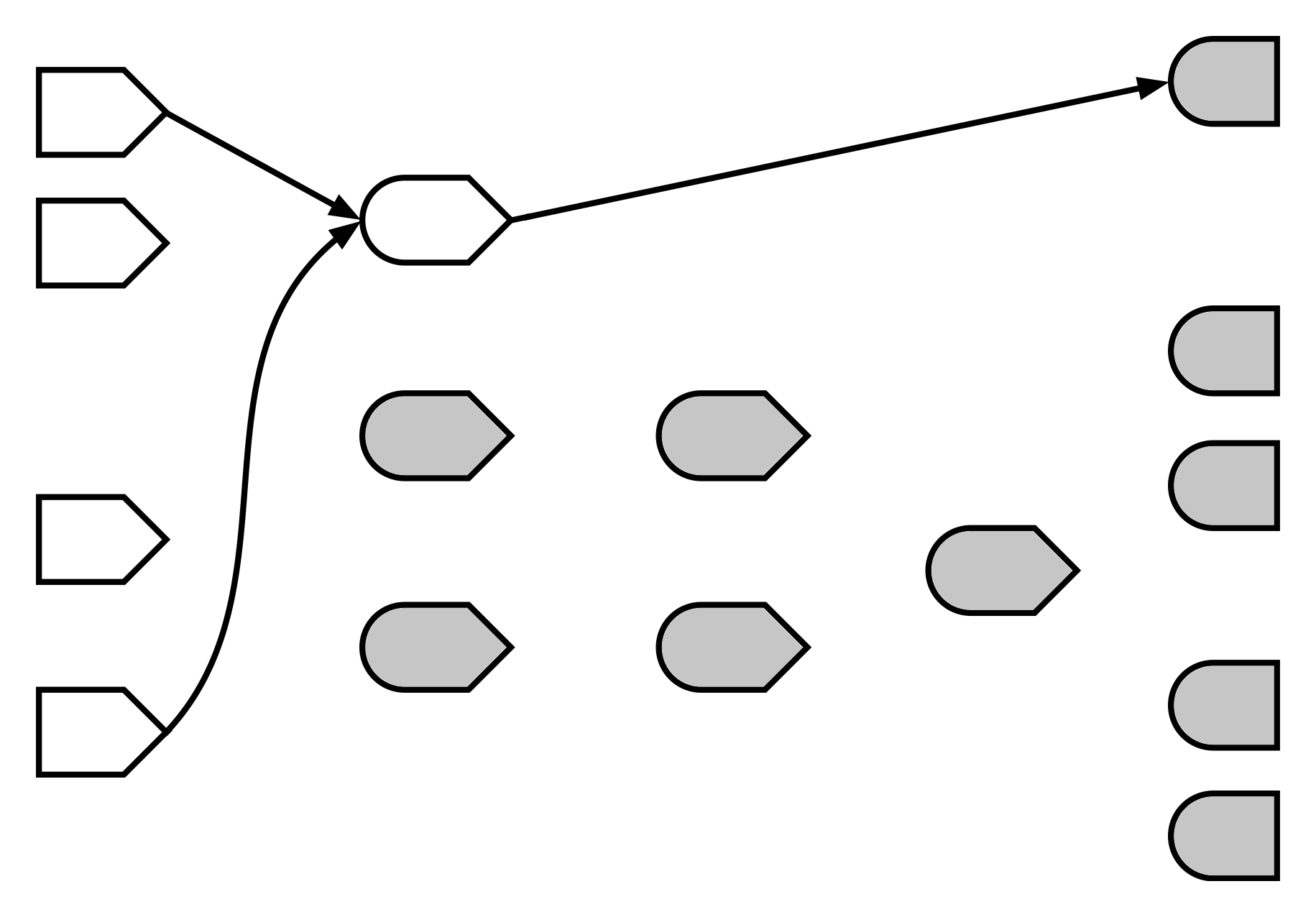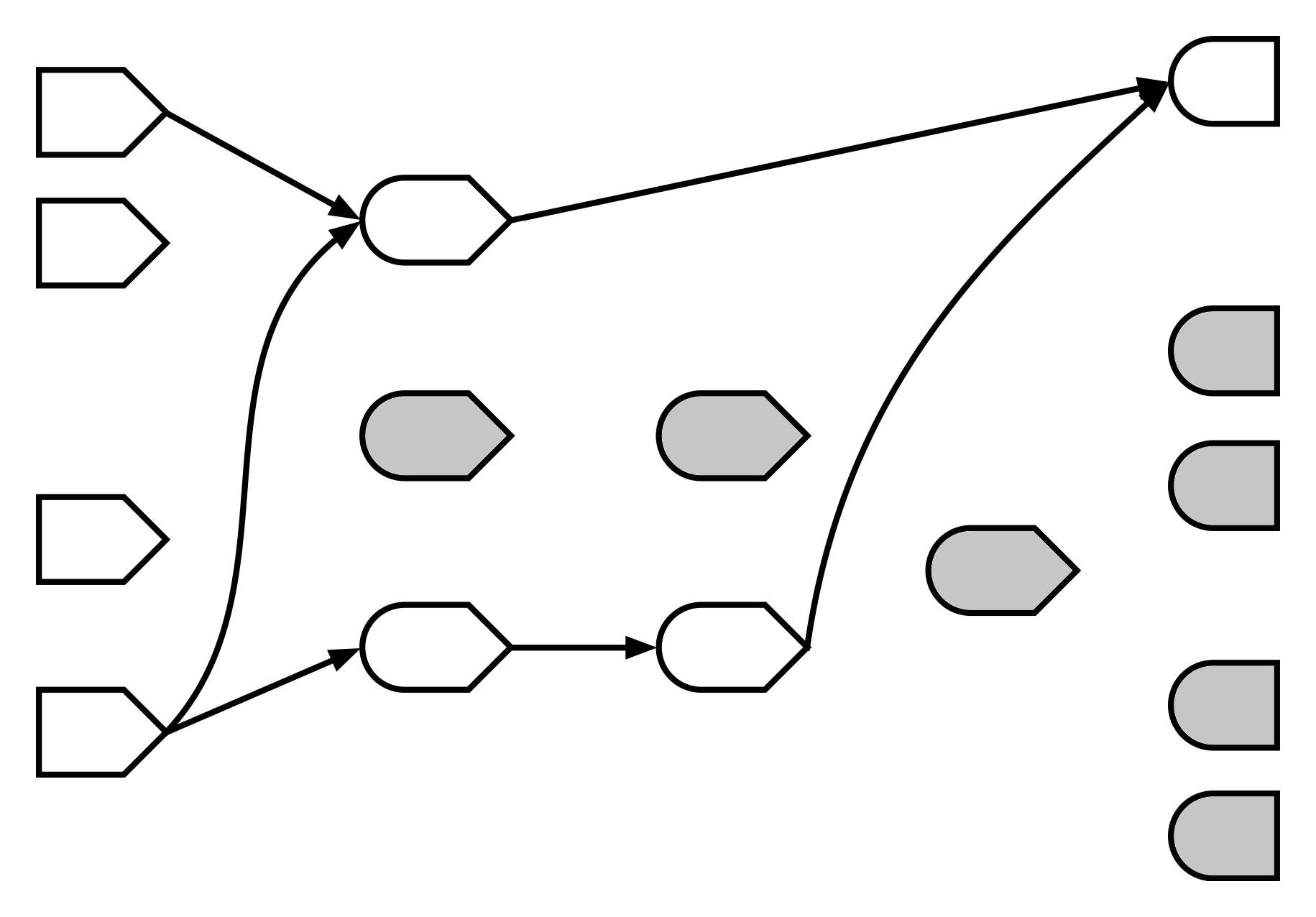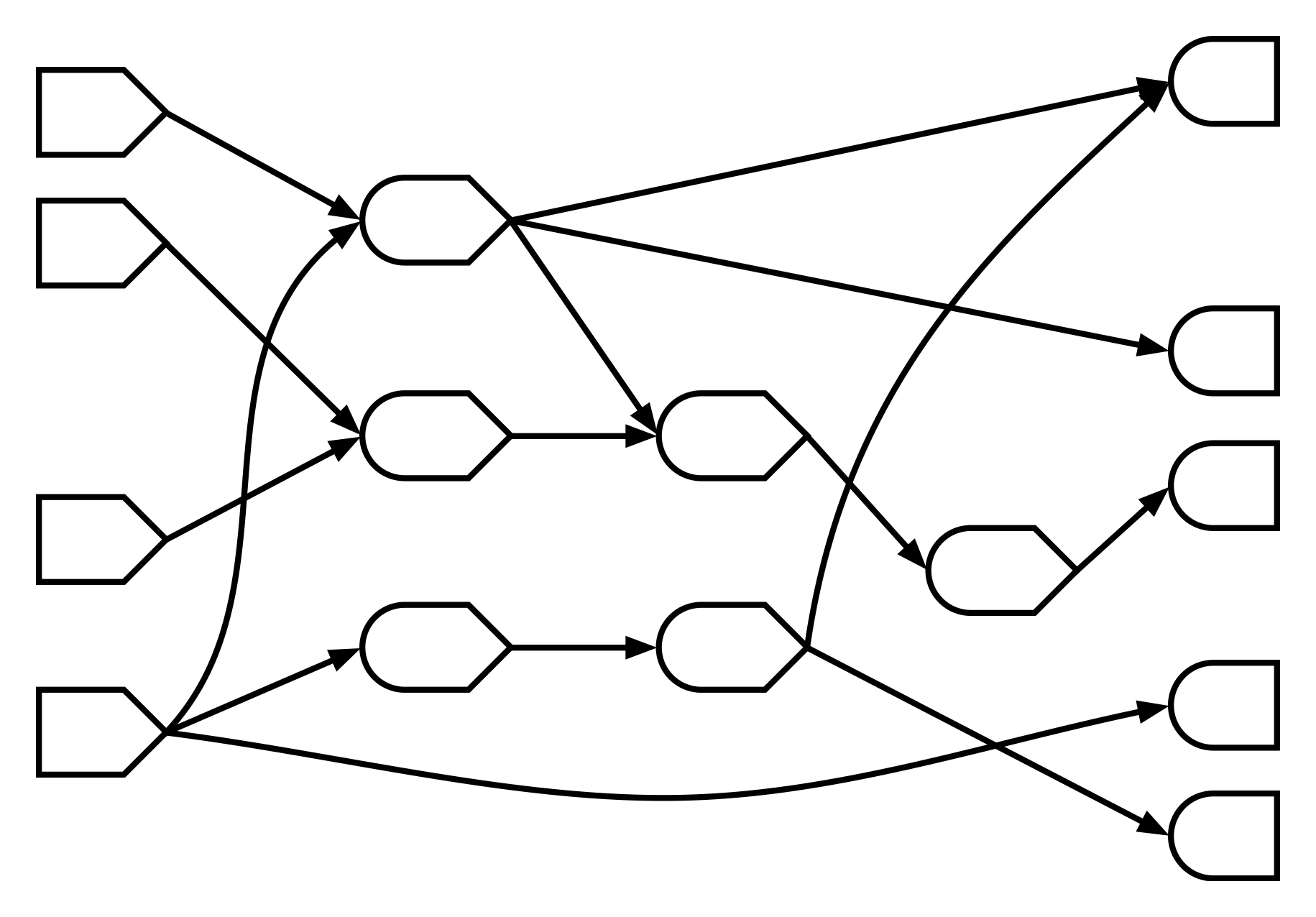# Warm up: Side effects

Functions can be executed for one or both of the following reasons:

1. You want its return value.
2. You want it to have some other effect.

These are (a bit misleadingly) called side effects. Any effect that is not the return value is a side effect.

# Functions with side effects

``````write.csv(...)

plot(cars)

print(x)

httr.POST(...)

alarm()``````

# More side effects

``````# Sets a variable in a parent environment
value <<- 10

# Loads into global env by default
source("functions.R")

# Modifies the global search list
library(dplyr)

# Only if foo is an env, ref class, or R6
foo\$bar <- TRUE``````

# NOT side effects (when inside a function)

``````# Modifying *local* variables
value <- 10

# Creating most kinds of objects
list(a = 1, b = 2)

# Data frames are pass-by-value in R so this is OK
dataset <- dataset %>% filter(count > 3)

# Most calculations
a + 1
summary(pressure)
lm(speed ~ dist, data = cars)
predict(wfit, interval = "prediction")``````

# Ehhh… Not side effects

``````# Reading from disk

# Making HTTP GET requests
httr.GET("https://api.example.com/data.json")

.Random.seed

# Modifying the random seed... ehhhhhh...
runif(10)``````

If executing your function/expression leaves the state of the world a little different than before it executed, it has side effects.

But if “what happens in `func`, stays in `func`” (besides the return value), then it doesn’t have side effects.

# Side effect quiz

For each function, write Yes if it has side effects, and No if not.

# Question 1

``````function(a, b) {
(b - a) / a
}``````

# Question 2

``````function(values) {
globalenv()\$values <- values
values
}``````

# Question 3

``````function() {
options(digits.secs = 6)
as.character(Sys.time())
}``````

# Question 4

``````function(df) {
df\$foo <- factor(df\$foo)
df
}``````

# Question 5

``````function() {
}``````

# Question 6

``````function(values) {
hist(values, plot = TRUE)
}``````

# Question 7

``````function() {
# Create temp file, and delete when function exits
filePath <- tempfile(fileext = ".png")

# Plot to the temp file as PNG image
png(filePath); plot(cars); dev.off()

# Return the contents of the temp file
}``````

1. No
2. Yes
3. Yes
4. No
5. No
6. Yes
7. Mostly no

Side effects make code harder to reason about, since order of execution of different side-effecty functions can matter (in non-obvious ways).

But we still need them. Without side effects, our programs are useless! (If a program executes but has no observable interactions with the world, you may as well have not executed it at all!)

# Reactive programming

1. Made it halfway through the tutorial. Has used `output` and `input`.
2. Made it entirely through the tutorial. Has used reactive expressions (`reactive()`).
3. Has used `observe()` and/or `observeEvent()`. Has written reactive expressions that depend on other reactive expressions. Has used `isolate()` properly.
4. Can say confidently when to use `reactive()` vs. `observe()`. Has used `invalidateLater`.
5. Writes higher-order reactives (functions that have reactive expressions as input parameters and return values).
6. Understands that reactive expressions are monads.

# Exercise 0

Open `Exercise_00.R` and complete the server function. Make the plot output show a simple plot of the first `nrows` rows of a built-in dataset.

You have 3 minutes!

Hint: `plot(head(cars, nrows))`

# Solution

``````output\$plot <- renderPlot({
})``````

# Anti-solution

``````observe({
output\$plot <- renderPlot(plot(df))
})``````

`output\$plot1 <- renderPlot(...)`

• DOESN’T mean: “Go update the output `"plot1"` with the result of this code.”
• DOES mean: “This code is the recipe that should be used to update the output `"plot1"`.”

# Reactive expressions

Expressions that are reactive (obviously)

• Expression: Code that produces a value
• Reactive: Detects changes in anything reactive it reads
``````function(input, output, session) {
# When input\$min_size or input\$max_size change, large_diamonds
# will be notified about it.
large_diamonds <- reactive({
diamonds %>%
filter(carat >= input\$min_size) %>%
filter(carat < input\$max_size)
})

# If that happens, large_diamonds will notify output\$table.
output\$table <- renderTable({
large_diamonds() %>% select(carat, price)
})``````
``````  ... continued ...

# Reactive expressions can use other reactive expressions.
mean_price <- reactive({
mean(large_diamonds()\$price)
})

# large_diamonds and mean_price will both notify output\$message
# of changes they detect.
output\$message <- renderText({
paste0(nrow(large_diamonds()), " diamonds in that range, ",
"with an average price of \$", mean_price())
})
}``````
``````function(input, output, session) {

# This DOESN'T work.
large_diamonds <- diamonds %>%
filter(carat >= input\$min_size) %>%
filter(carat < input\$max_size)

output\$table <- renderTable({
large_diamonds %>% select(carat, price)
})
}``````

`large_diamonds` would only be calculated once, as the session starts (i.e. as the page first loads in a browser).

# Exercise 1

Open up the file `Exercise_01.R`.

There’s a new `tableOutput("table")` in ui.R. Have it show the same data frame that is being plotted, using `renderTable`.

Make sure that the `head()` operation isn’t performed more than once for each change to `input\$nrows`.

You have 5 minutes.

# Solution

``````function(input, output, session) {

df <- reactive({
})

output\$plot <- renderPlot({
plot(df())
})

output\$table <- renderTable({
df()
})
}``````

# Anti-solution 1

``````function(input, output, session) {

values <- reactiveValues(df = cars)
observe({
})

output\$plot <- renderPlot({
plot(values\$df)
})

output\$table <- renderTable({
values\$df
})
}``````

# Anti-solution 2

``````function(input, output, session) {

df <- cars
observe({
})

output\$plot <- renderPlot({
plot(df)
})

output\$table <- renderTable({
df
})
}``````

# Exercise 2

Open up the file `Exercise_02.R`.

This is a working app–you can go ahead and run it. You choose variables from the iris (yawn) data set, and on various tabs it shows information about the selected variables and fits a linear model.

The problem right now, is that each of the four outputs contains copied-and-pasted logic for selecting out your chosen variables, and for building the model. Can you refactor the code so it’s more maintainable and efficient?

You have 5 minutes.

# Solution

``````selected <- reactive({
iris[, c(input\$xcol, input\$ycol)]
})

model <- reactive({
lm(paste(input\$ycol, "~", input\$xcol), selected())
})``````